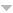Publications

Found 2290 results
Author Title Type [ Year]
Filters: Filter is   [Clear All Filters]
1992
On the power semigroup of a finite semigroup. Portugal. Math.. 1992;49:295-331.Edit
Rational languages and inverse monoid presentations. Internat. J. Algebra Comput.. 1992;2:187-207.
Semigroups whose idempotents form a subsemigroup. Math. Proc. Cambridge Philos. Soc.. 1992;111:241-253.Edit
Some classes of discrete transforms that are generated by matrix linear operators. Vests\=ı Akad. Navuk Belarus\=ı Ser. F\=ız. Mat. Navuk. 1992:20-25, 123.
Some generalizations of the Laplace convolution. Mat. Fiz. Neline\uın. Mekh.. 1992:8-12.
1991
Algebraic varieties characterizing matroids and oriented matroids. Adv. Math.. 1991;87:160-185.Edit
The Arrhenius plot of a physiological rate process is never linear. Ciência e Cultura. 1991;43(5):363-369.Edit
On direct product decompositions of finite \$\scr J\$-trivial semigroups. Internat. J. Algebra Comput.. 1991;1:329-337.Edit
On finite simple semigroups. Proc. Edinburgh Math. Soc. (2). 1991;34:205-215.Edit
The generalizations of integral analog of the Leibniz rule on the \$G\$-convolutions. Extracta Math.. 1991;6:119-122.Edit
Generalizations of the Leibniz rule to integral convolutions. Dokl. Akad. Nauk BSSR. 1991;35:111-115, 188.Edit
Generating operators and convolutions for some integral transformation. Dokl. Akad. Nauk BSSR. 1991;35:773-776, 860.Edit
Geometric conditions for local controllability. J. Differential Equations. 1991;89:388-395.
Implicit operations on finite \$\scr J\$-trivial semigroups and a conjecture of I. Simon. J. Pure Appl. Algebra. 1991;69:205-218.Edit
Integral convolutions for \$H\$-transformations. Izv. Vyssh. Uchebn. Zaved. Mat.. 1991:72-79.Edit
Integral convolutions of Laplace type for \$G\$-transforms. Vests\=ı Akad. Navuk BSSR Ser. F\=ız.-Mat. Navuk. 1991:11-16, 123.
On iterated semidirect products of finite semilattices. J. Algebra. 1991;142:239-254.Edit
The least squares problems and orthogonal polynomials. In: Orthogonal polynomials and their applications (Erice, 1990). Vol 9. Baltzer, Basel; 1991. 2. p. 217-222p. (IMACS Ann. Comput. Appl. Math.; vol 9).Edit
Local controllability along a reference trajectory. J. Math. Anal. Appl.. 1991;158:55-62.
Local controllability of scalar input systems on \$3\$-manifolds. Systems Control Lett.. 1991;16:349-355.
On Mason's extension of the Erdős-Rényi law of large numbers. Statist. Probab. Lett.. 1991;11:43-47.Edit
On the membership problem for pseudovarieties of commutative semigroups. Semigroup Forum. 1991;42:47-51.Edit
Recent developments in the theory of implicit operations. In: Monoids and semigroups with applications (Berkeley, CA, 1989). World Sci. Publ., River Edge, NJ; 1991. 1. p. 105-117p. Edit
Reduction of Hamiltonian systems with symmetry. J. Differential Equations. 1991;94:95-111.
Semigrupos finitos e álgebra universal Universidade de São Paulo, Instituto de Matemática e Estatí stica, São Paulo 1991.Edit